# Clock Tower

What angle is betwenn hands on Clock Tower when show 17 hours and 35 minutes?

x =  42.5 °

### Step-by-step explanation: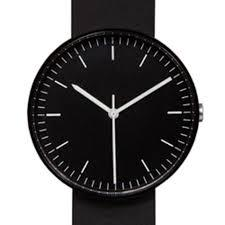Did you find an error or inaccuracy? Feel free to write us. Thank you!Tips to related online calculators
Do you want to convert velocity (speed) units?
Do you want to convert time units like minutes to seconds?

#### You need to know the following knowledge to solve this word math problem:

We encourage you to watch this tutorial video on this math problem:

## Related math problems and questions:

• Clock hands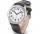The hands-on clock shows the time 12 hours and 2 minutes. Calculate the size of an acute angle between clock hands three hours later.
• HandsThe clock shows 12 hours. After how many minutes will angle between the hour and minute hand 90°? Consider the continuous movement of both hands hours.
• Time clockWhat are the angle of the hour hand and the minute hand if it is 0:40?
• RPMAn electric motor makes 3,000 revolutions per minutes. How many degrees does it rotate in one second?
• Clock losing time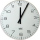A clock is showing 9:30 am. If the clock is 5 minutes slow, what would have been the correct time?
• Minute handWhat is the distance the minute hand of the clock travels in 12 minutes, if the diameter of the clock is 30 cm and the hand extends to a distance of 2 cm from the edge of the clock?
• Two trains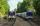Two trains go at each other. First at speed 40 km/h and the second at speed 60 km/h. At what distance will be 30 minutes before they will meet?
• The big clock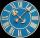The big clock hands stopped at a random moment. What is the probability that: a) a small hand showed the time between 1:00 and 3:00? b) the big hand was in the same area as a small hand in the role of a)? c) did the hours just show the time between 21:00
• ClockHow many times a day hands on a clock overlap?
• Fog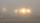The car started in fog at a speed of 30 km/h. After a 12-minute drive, the fog dissipated, and the driver drove the next 12 minutes distance, 17 km. On the last 17 km long again, the driving conditions deteriorated, and the driver drove at a speed of 51 k
• A clockA clock was set right at 6:00 AM. If it gains 3 1/2 minutes per hour, what time will it show at 6:00 PM on the same day? Show your solution
• Quarter of an hourIf the clock showed a quarter of an hour more, it would be 10 minutes to 10 hours. How many hours do they show?
• The Nürburgring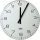The Nürburgring in Germany is a racetrack that hosts racing events all year. Two of the fastest laps ever driven on the track are 6 minutes 47.30 seconds and 6 minutes 52.01 seconds. How much faster is the 6 minutes 47.30 seconds time than the 6 minutes 5
• Truck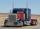Truck went for 4 hours and 30 minutes. If truck increase speed by 11 km/h, went same distance in 2 hours. Calculate this distance.
• Rotary motionFind the orbital time, rotational frequency, and angular rate of the second and minute hands on the watch.
• Little hand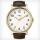What angle shifted little hand on the clock after one hour and 38 minutes?
• Walking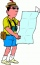Lucie can walk about 3 4/5 miles each hour. How far can she walk in 2 hours 45 minutes?